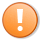# Quick-tip 32 | Creating a function to regulate the number of drawing objects

Quick tip tutorial 32 demonstrates the use of a function that, when called by an analysis technique that creates drawing objects, ensures that there are never more than the input number plus one drawing objects created by that analysis technique, on the chart.

The function is called using the following syntax: Condition1 = QuickTip32( Num ); where Num is an integer, for example,

`Condition1 = QuickTip32( 4999 );`

The example indicator and function can be downloaded by Gold Pass members, below.

This quick-tip follows the same subject area as quick tip 31, i.e. managing the number of drawing objects drawn on a chart. To demonstrate the function I have created a simple indicator that draws an ellipse, a rectangle, a vertical line, a horizontal line, a trendline and some text on each bar. The indicator calls the function and the function makes sure that there are no more drawing objects of a certain type than its input value.

This quick-tip uses the new TradeStation drawing objects, as opposed to legacy drawing objects. It does not apply to MultiCharts users.

The function works as follows. It the number of a particular drawing object is over the input value it deletes the oldest drawing object of the same type, using the following syntax:

`DrawingObjects.Delete( ( DrawingObjects.Items[Ctr] astype Vector ) astype DrawingObject );`

DrawingObjects.Items[Ctr] astype Vector is a vector that contains all the drawing objects of type Ctr. In the function I use the following types with the corresponding reference number:

• TrendLineCreatedByAnalysisTechnique 1
• TextCreatedByAnalysisTechnique 4
• RectangleCreatedByAnalysisTechnique 7
• HorizontalLineCreatedByAnalysisTechnique 10
• VerticalLineCreatedByAnalysisTechnique 13
• EllipseCreatedByAnalysisTechnique 16

So DrawingObjects.Items astype Vector is a vector of text objects created by an analysis technique.

The drawing object in the zero position of the vector is the oldest. Notice the use of astype in the syntax.

### Quick-tip 32 videoThis content is for members only.